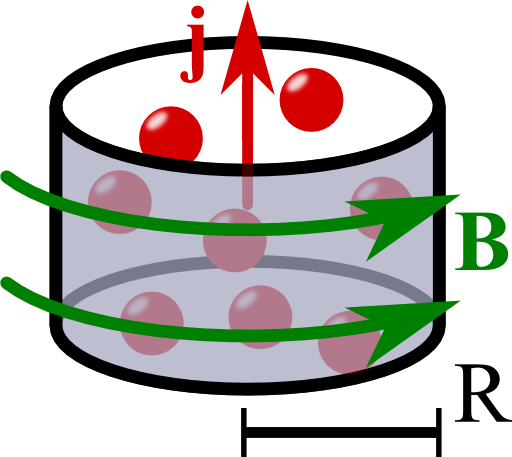The Magnetic Field of an Infinite WireOne of the basic problems of magnetostatics is the infinite wire. Using the several symmetries of the current distribution we will be able to not only find the magnetic field - we will also verify the "right-hand rule".

Problem StatementCalculate the magnetic field of an infinitely long wire with a uniform current distribution$\mathbf{j}\left(\mathbf{r}\right) = \begin{cases}j_{0} & \rho\leq R\\0 & \mathrm{else}\end{cases}\mathbf{e}_{z}$in the following way:

1. Explain why the magnetic field for the given current distribution only depends on $$\rho$$ (cylindrical coordinates), i.e. why $$\mathbf{B}\left(\mathbf{r}\right)=\mathbf{B}\left(\rho\right)$$.
2. Additional - Verify the “right-hand-rule”: - if a current distribution is generally given by $$j\left(\rho\right)\mathbf{e}_{z}$$ (translation and rotation symmetry), show that the magnetic field can only have an angular   component $$\varphi$$.
3. Use the integral form of Ampère's law to calculate the magnetic field everywhere.

Note that we use mostly differential methods to calculate the fields everywhere in this problem. In "The Magnetic Field due to a Thin Infinite Wire" we use the Biot-Savart law (integration!) to determine the magnetic field. Nevertheless, our general solution here is also valid with respect to thin wires if we let $$R\rightarrow 0$$.